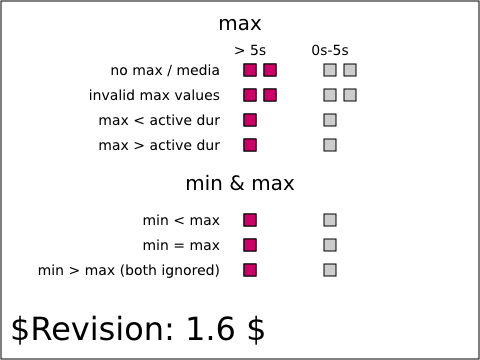Tests that contain the draft-watermark are under development and may be incorrectly testing a feature.

# animate-elem-66-t.svg## Operator Script

Run the test and observe it for at least six seconds. No interaction required.

## Pass Criteria

The test passes if for the first five seconds after the document loads, the red squares in each row (two in the first two rows, and one each in the remaining rows) are in the right column, and after the five seconds, they all move to the left column.

## Test Description

This tests performs basic tests on the max attribute and on combinations of the min and max attributes. The test is based on the SMIL specification at: http://www.w3.org/TR/smil20/smil-timing.html#Timing-MinMax.

Each row in the test shows different rectangles subject to <set> animations with different configurations with regards to the max and min attributes. For each row, the animation should be active during the first 5 seconds of the animations where the red rectangle should show in the right column. At five seconds into the animation, all the rectangles should move to their left position.

On the first row, the <set> animation has a (0s <= t < 5s) active duration and no max attribute so the actual active duration is (0s <= t < 5s). The first row shows a second rectangle with a <set> animation with the same configuration except that the max attribute value is set to 'media'. Because the <set> element does not define a media, the behavior should be as if the attribute was not specified.

On the second row, the <set> animation has a (0s <= t < 5s) active duration and a min attribute set to '-6s' for the first rectangle and to 'foo' for the second one. These values are invalid for max and, as per the specification, the behavior should be as if the attribute was not specified. Consequently, the behavior is as for the previous row and the actual active duration is (0s <= t < 5s).

On the third row, the <set> animation has a (0s <= t < 8s) initial active duration and a max attribute set to '5s'. The max value is less than the active duration, so the max attribute constrains the active duration to (0s <= t < 5s).

On the fourth row, the <set> animation has a (0s <= t < 5s) initial active duration, an indefinite simple duration (dur is set to indefinite) and a max attribute set to '8s'. Because the initial active duration is less than the max attribute the active duration is not constrained and is unchanged at (0s <= t < 5s).

On the fifth row, the <set> animation has a (0s <= t < indefinite) initial active duration, a min of 2s and a max of 5s. Because the min value is less than the max value, both apply and the computed active duration is (0s <= t < 5s).

On the sixth row, the <set> animation has a (0s <= t < indefinite) initial active duration, a min of 5s and a max of 5s. Because the min value is equal to the max value, both apply and the computed active duration is (0s <= t < 5s).

On the seventh row, the <set> animation has a [0s, 5s[[ initial active duration, a min of 8s and a max of 2s. Because the min value is greater than the max value, both are ignored and the computed active duration is [0s, 5s[.